How to Use SPSS to Conduct a Thorough Multiple Linear Regression analysis

 We provide SPSS Help for Students, at any level! Get professional graphs, tables, syntax, and fully completed SPSS projects, with meaningful interpretations and write up, in APA or any format you prefer. Whether it is for a Statistics class, Business Stats class, a Thesis or Dissertation, you'll find what you are looking for with us Our service is convenient and confidential. You will get excellent quality SPSS help for you. Our rate starts at \$35/hour. Free quote in hours. Quick turnaround!

Results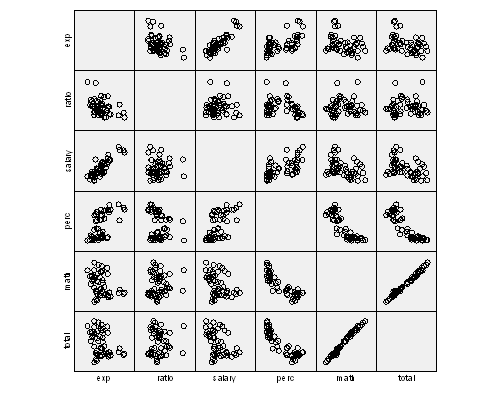Descriptive Statistics: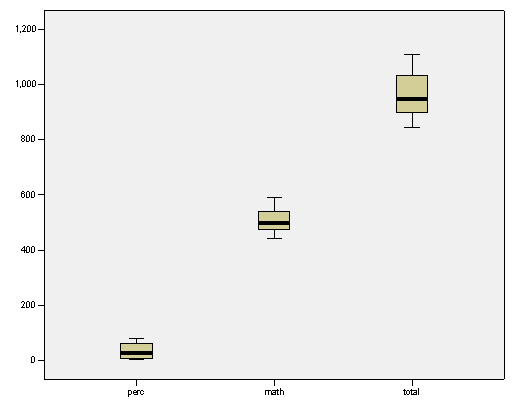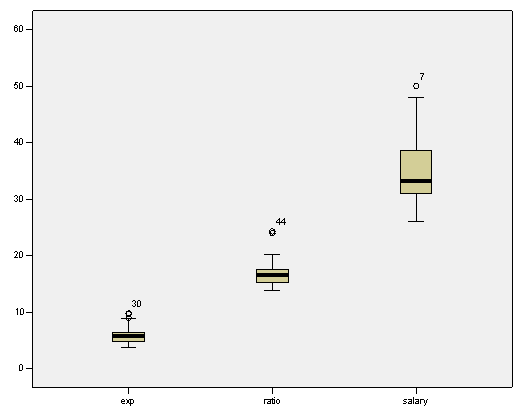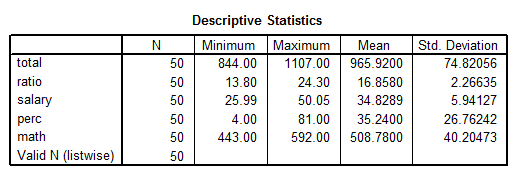Regression Results:• The table above shows that the correlation coefficient is R = 0.995, and the adjusted R2 is 0.989, which indicates that 98.9% of the variation in Total is explained by this model• The table above shows that the model is significant overall (p = 0.000), this means that at least one variable in this model is significant. This is not surprising considering the type of scatterplot found.$Total = 113.505 + 1.694Math - 0.268Perc$

Regression Assumptions: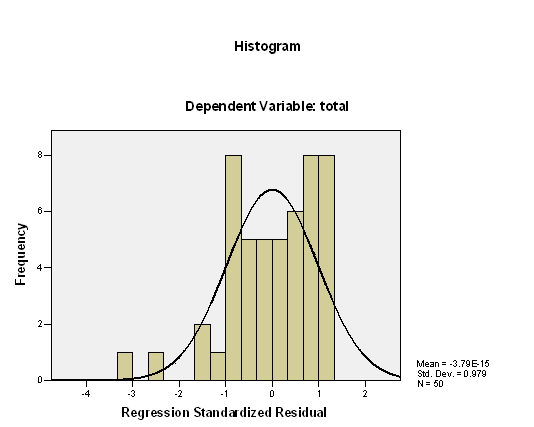Conclusions

$Total=113.505+1.694Math-0.268Perc$
• Alternative Model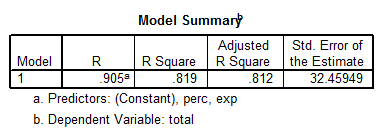The model is
$Total=993.832+12.287Exp-2.851Perc$